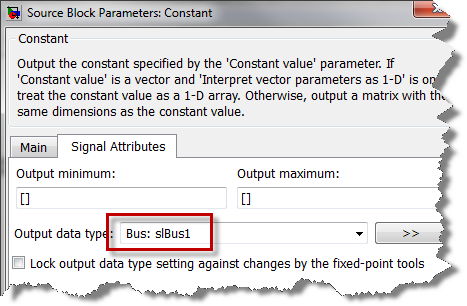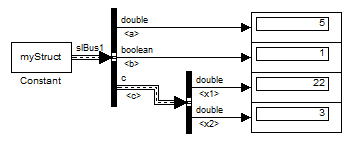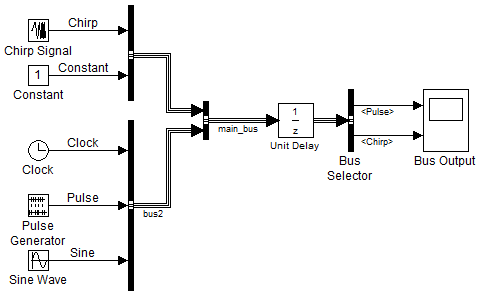Initializing buses using a MATLAB Structure

Did you notice that since R2010a it is possible to initialize buses with a MATLAB structure? If you were not aware, here is how it works.

Starting from a Structure

Let's say I have a structure in the MATLAB workspace and I would like to make that a bus signal in Simulink. I can use Simulink.Bus.createObject to create a bus object:

myStruct.a = 5;
myStruct.b = true;
myStruct.c.x1 = 22;
myStruct.c.x2 = 3;

Then I can configure a Constant block to use this bus object as data type and to use myStruct as value.Starting from a model

Let's say I have the following model where a bus signal is going through a Unit Delay block. I want to specify different initial values for the elements of the bus.In that case I can use the handle to a port of the Unit Delay block as input to Simulink.Bus.createMATLABStruct. This will create a MATLAB structure with the same hierarchy as the bus, filled with zeros. I can then overwrite fields of this structure as needed and use it as the initial value of the Unit Delay.

portHandle = get_param(gcb,'PortHandles');
initStruct.bus2.Pulse = 5;
initStruct.signal1.Chirp = 3;

Requirements to Use Bus Signal Initialization

To enable bus signal initialization, you need to set two Configuration Parameter diagnostics:

Even if you don't need to initialize buses, I always recommend using those setting. They help making your models easier to understand and improve the consistency of simulation results.